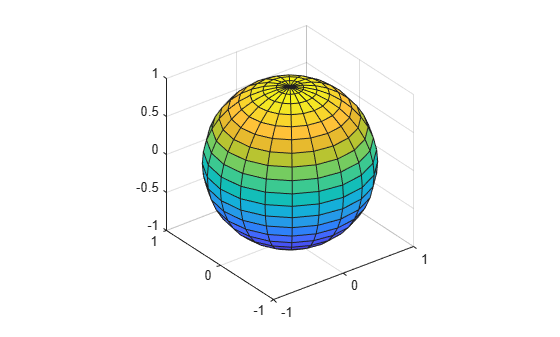# sphere

## 语法

``[X,Y,Z] = sphere``
``[X,Y,Z] = sphere(n)``
``sphere(___)``
``sphere(ax,___)``

## 说明

``[X,Y,Z] = sphere` 返回球面的 x、y 和 z 坐标而不对其绘图。返回的球面的半径等于 `1`，由 20×20 个面组成。 该函数以三个 `21`×`21` 矩阵形式返回 x、y 和 z 坐标。要使用返回的坐标绘制球面，请使用 `surf` 或 `mesh` 函数。`

``[X,Y,Z] = sphere(n)` 返回半径等于 `1` 且包含 `n`×`n` 个面的球面的 x、y 和 z 坐标。该函数以三个 `(n+1)`×`(n+1)` 矩阵形式返回 x、y 和 z 坐标。`

``sphere(___)` 绘制球面而不返回坐标。请将此语法与上述语法中的任何输入参数结合使用。`

``sphere(ax,___)` 将图形绘制到 `ax` 指定的坐标区中，而不是当前坐标区中。指定坐标区作为第一个输入参数。`

## 示例

```sphere axis equal````X``Y``Z` 定义为单位球面的坐标。

`[X,Y,Z] = sphere;`

```surf(X,Y,Z) axis equal``````hold on r = 5; X2 = X * r; Y2 = Y * r; Z2 = Z * r; surf(X2+5,Y2-5,Z2)``````tiledlayout(2,2); ax1 = nexttile; sphere(ax1); axis equal title('20-by-20 faces (Default)') ax2 = nexttile; sphere(ax2,50) axis equal title('50-by-50 faces') ax3 = nexttile; sphere(ax3,100) axis equal title('100-by-100 faces')```